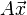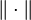# Spectral norm

## Definition

### For a real matrix

The spectral norm of a$n \times n$ square matrix$A$ with real entries is defined in the following equivalent ways:

1. It is the maximum of the Euclidean norms of vectors$A\vec{x}$ where$\vec{x}$ is on the unit sphere, i.e., has Euclidean norm 1.
2. It is the maximum, over all nonzero vectors$\vec{x} \in \R^n$, of the quotients$\frac{\| A\vec{x} \|}{\| \vec{x} \|}$ where$\| \cdot \|$ denotes the Euclidean norm.
3. It is the largest singular value of$A$, or equivalently, it is the square root of the largest eigenvalue of the product$AA^T$.

### For a complex matrix

Fill this in later